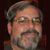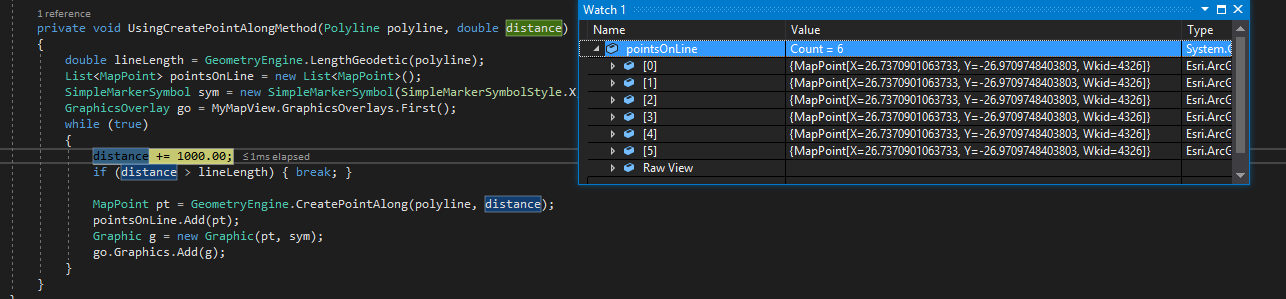# Create points at equal distance along a polyline

2265
4
02-18-2019 03:38 AMNew Contributor II

Hi team,

how to get the points only which were located for every 2 kms on a poly line?

I tried Densify method to create new points along the poly line, but after densify, it returns the whole points list(newly created + old points). I can't figure out which was plotted at exactly 2 kms and which was not. So please suggest me a way to achieve this.

Tags (3)
1 Solution

Accepted SolutionsbyEsri Contributor

The problem is that your line is measured in degrees of longitude/latitude and the value you're passing in is for meters. The code is reading the distance of "1000.0" as 1000 degrees rather than 1 KM. Try projecting the line to a projected coordinate system for your area (looks like South Africa?). Be sure you know the units for the projected line, it might be feet instead of meters!

Here's an updated example that projects the line before processing, and also "unprojects" the result points back to Lat/Long (warning: this code hasn't been tested much😞

``````double distance = 0.0;
Polyline projectedLine = (Polyline)GeometryEngine.Project(_originalPolyline, new SpatialReference(102472));
double lineLength = GeometryEngine.LengthGeodetic(projectedLine);
List<MapPoint> pointsOnLine = new List<MapPoint>();
SimpleMarkerSymbol sym = new SimpleMarkerSymbol(SimpleMarkerSymbolStyle.X, Color.Yellow, 16);
GraphicsOverlay go = MyMapView.GraphicsOverlays.First();
while (true)
{
distance += 1000.0;
if(distance > lineLength) { break; }
MapPoint pt = GeometryEngine.CreatePointAlong(projectedLine, distance);
MapPoint latLongPoint = (MapPoint)GeometryEngine.Project(pt, SpatialReferences.Wgs84);
Graphic g = new Graphic(latLongPoint, sym);
}‍‍‍‍‍‍‍‍‍‍‍‍‍‍‍‍``````

(Also, check out the "ConvertTo" and "ConvertFrom" methods on the LinearUnits to convert between a variety of units. Works for angles and areas too: Esri.ArcGISRuntime.Geometry.LinearUnits.Kilometers.ConvertFrom(otherUnit, val))

4 RepliesbyEsri Contributor

Give the code below a try. It increments the distance to create a point along the line. When it reaches the end of the line it stops. It assumes the line is measured in meters, so uses 2000 to represent 2 km along the line.

``````double distance = 0.0;
double lineLength = GeometryEngine.LengthGeodetic(_originalPolyline);
List<MapPoint> pointsOnLine = new List<MapPoint>();
SimpleMarkerSymbol sym = new SimpleMarkerSymbol(SimpleMarkerSymbolStyle.X, Color.Yellow, 16);
GraphicsOverlay go = MyMapView.GraphicsOverlays.First();
while (true)
{
distance += 2000.0;
if(distance > lineLength) { break; }

MapPoint pt = GeometryEngine.CreatePointAlong(_originalPolyline, distance);
Graphic g = new Graphic(pt, sym);
}‍‍‍‍‍‍‍‍‍‍‍‍‍‍‍‍‍‍‍‍‍‍‍‍‍‍‍‍‍‍‍‍‍‍‍‍‍‍‍‍‍‍‍``````

HTH!New Contributor II

I tried using your above implementation.

I have a poly line with 7 kms(approx) of length which i'm extracting from a shape file by creating a feature layer, now by following your code i want to create points along poly line at every 1km distance. below is the code

``````private void UsingCreatePointAlongMethod(Polyline polyline, double distance)
{
double lineLength = GeometryEngine.LengthGeodetic(polyline);
List<MapPoint> pointsOnLine = new List<MapPoint>();
SimpleMarkerSymbol sym = new SimpleMarkerSymbol(SimpleMarkerSymbolStyle.X, Color.Yellow, 16);
GraphicsOverlay go = MyMapView.GraphicsOverlays.First();
while (true)
{
distance += 1000.0;
if (distance > lineLength) { break; }
MapPoint pt = GeometryEngine.CreatePointAlong(polyline, distance);
Graphic g = new Graphic(pt, sym);
}
}‍‍‍‍‍‍‍‍‍‍‍‍‍‍‍‍‍‍‍‍‍‍‍‍‍‍‍‍‍‍‍‍``````

but the code returns the same point every time and it's always pointing at the starting of the poly line. here i'm also attaching the screenshots.byEsri Contributor

The problem is that your line is measured in degrees of longitude/latitude and the value you're passing in is for meters. The code is reading the distance of "1000.0" as 1000 degrees rather than 1 KM. Try projecting the line to a projected coordinate system for your area (looks like South Africa?). Be sure you know the units for the projected line, it might be feet instead of meters!

Here's an updated example that projects the line before processing, and also "unprojects" the result points back to Lat/Long (warning: this code hasn't been tested much😞

``````double distance = 0.0;
Polyline projectedLine = (Polyline)GeometryEngine.Project(_originalPolyline, new SpatialReference(102472));
double lineLength = GeometryEngine.LengthGeodetic(projectedLine);
List<MapPoint> pointsOnLine = new List<MapPoint>();
SimpleMarkerSymbol sym = new SimpleMarkerSymbol(SimpleMarkerSymbolStyle.X, Color.Yellow, 16);
GraphicsOverlay go = MyMapView.GraphicsOverlays.First();
while (true)
{
distance += 1000.0;
if(distance > lineLength) { break; }
MapPoint pt = GeometryEngine.CreatePointAlong(projectedLine, distance);
MapPoint latLongPoint = (MapPoint)GeometryEngine.Project(pt, SpatialReferences.Wgs84);
Graphic g = new Graphic(latLongPoint, sym);May God Bless You.